Centre Of Mass NAT Level - 1

# Centre Of Mass NAT Level - 1

Test Description

## 10 Questions MCQ Test Topic wise Tests for IIT JAM Physics | Centre Of Mass NAT Level - 1

Centre Of Mass NAT Level - 1 for IIT JAM 2023 is part of Topic wise Tests for IIT JAM Physics preparation. The Centre Of Mass NAT Level - 1 questions and answers have been prepared according to the IIT JAM exam syllabus.The Centre Of Mass NAT Level - 1 MCQs are made for IIT JAM 2023 Exam. Find important definitions, questions, notes, meanings, examples, exercises, MCQs and online tests for Centre Of Mass NAT Level - 1 below.
Solutions of Centre Of Mass NAT Level - 1 questions in English are available as part of our Topic wise Tests for IIT JAM Physics for IIT JAM & Centre Of Mass NAT Level - 1 solutions in Hindi for Topic wise Tests for IIT JAM Physics course. Download more important topics, notes, lectures and mock test series for IIT JAM Exam by signing up for free. Attempt Centre Of Mass NAT Level - 1 | 10 questions in 30 minutes | Mock test for IIT JAM preparation | Free important questions MCQ to study Topic wise Tests for IIT JAM Physics for IIT JAM Exam | Download free PDF with solutions
 1 Crore+ students have signed up on EduRev. Have you?
*Answer can only contain numeric values
Centre Of Mass NAT Level - 1 - Question 1

### A block of mass 0.50 kg is moving with a speed of 2.00 ms–1 on a smooth surface. It strikes another mass of 1.00 kg and then they move together as a single body. The energy loss (in Joule) during the collision is :

Detailed Solution for Centre Of Mass NAT Level - 1 - Question 1

pi = pf  (momentum conservation)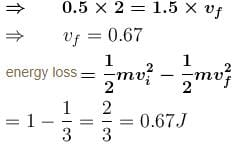*Answer can only contain numeric values
Centre Of Mass NAT Level - 1 - Question 2

### A force time graph for a linear motion is shown in figure where the segments are circular. The linear momentum gained between 0 to 8 second is :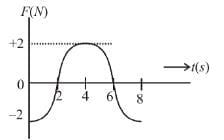Detailed Solution for Centre Of Mass NAT Level - 1 - Question 2

Change in momentum = area F-t graph = 0

*Answer can only contain numeric values
Centre Of Mass NAT Level - 1 - Question 3

### A bullet of mass  m = 50gm strikes (Δt ≈ 0) a sand bag of mass M = 5 kg hanging from a fixed point with a horizontal velocity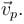If bullet sticks to the sand bag then the ratio of final and initial kinetic energy of the bullet is 10-α. Find the value of α:

Detailed Solution for Centre Of Mass NAT Level - 1 - Question 3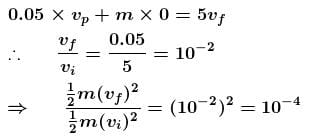*Answer can only contain numeric values
Centre Of Mass NAT Level - 1 - Question 4

A shell of mass 100 kg is fired horizontally from a canon with a mass of 5 × 103kg. The kinetic energy of the shell at the end of the barrel is 7.5 × 106 J. The kinetic energy imparted to the cannon by the recoil is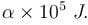Find the value of  α:

Detailed Solution for Centre Of Mass NAT Level - 1 - Question 4

m = 100 kg
M = 5×103 kg
Initially system is at rest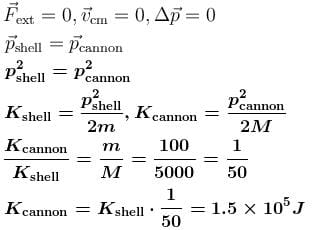*Answer can only contain numeric values
Centre Of Mass NAT Level - 1 - Question 5

Two masses of 1gm and 4gm are moving with equal kinetic energy. Find the Ratio of the magnitude of their linear momentum :

Detailed Solution for Centre Of Mass NAT Level - 1 - Question 5

KE = 1/2 m*v2

Momentum (p) = m*v

Set each of the 1 gram and 4 grams KE to 8:

KE = 1/2 *1* (4)= 8

KE = 1/2 *4* (2)= 8

The 1 gram takes 4 m/s to achieve KE of 8.

The 4 gram takes 2 m/s to achieve KE of 8.

Therefore, the ratio of their linear momentum (m*v) is:

1*4/4*2 =  4/8 = 1/2

*Answer can only contain numeric values
Centre Of Mass NAT Level - 1 - Question 6

A ball of mass 50gm is dropped from a height = 10m. It rebounds losing 75 percent of its kinetic energy. If it remains in contact with the ground for  Δt = 0.01 sec., the impulse of the impact force (in N-s)  is : (take =10 m/s2)

Detailed Solution for Centre Of Mass NAT Level - 1 - Question 6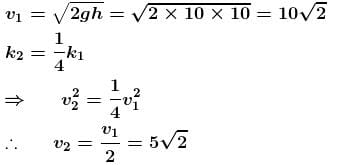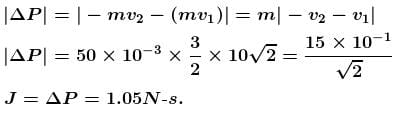*Answer can only contain numeric values
Centre Of Mass NAT Level - 1 - Question 7

A ball collides with an inclined plane of inclination θ after falling through a distance h. If it moves horizontally just after the impact, the coefficient of restitution is, when θ = 30: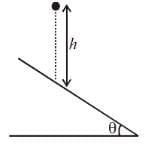Detailed Solution for Centre Of Mass NAT Level - 1 - Question 7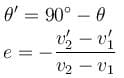where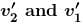are velocities components of wedge and ball along the impulsive force after collision and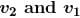are velocities components of wedge and ball along the impulsive force before collision
Wedge is at rest so,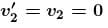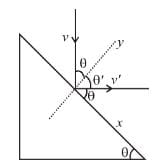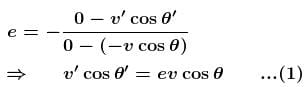conserving the linear momentum in x-direction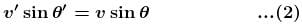On dividing equation (2) by (1)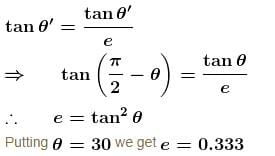*Answer can only contain numeric values
Centre Of Mass NAT Level - 1 - Question 8

A body of mass = 3.513 kg is moving along the x-axis with a speed of  5.00 m/s–1. The magnitude of its momentum (in kgms –1) is recorded as :

Detailed Solution for Centre Of Mass NAT Level - 1 - Question 8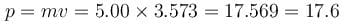As the lowest no. of significant figure is 3.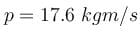*Answer can only contain numeric values
Centre Of Mass NAT Level - 1 - Question 9

Three particles of mass 20gm, 30gm and 40gm are initially moving along the positive direction of the three coordinate axes respectively with the same velocity of 20 cm/s. If due to their mutual interaction, the first particle comes to rest, the second acquires a velocity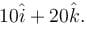What is the magnitude of velocity (in cm/s) of the third particle?

Detailed Solution for Centre Of Mass NAT Level - 1 - Question 9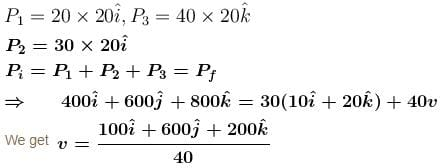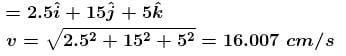*Answer can only contain numeric values
Centre Of Mass NAT Level - 1 - Question 10

Two particles of mass 1kg and 0.5kg are moving in the same direction with speed of 2 m/s and 6 m/s respectively on a smooth horizontal surface. The speed of centre of mass of the system in m/s is :

Detailed Solution for Centre Of Mass NAT Level - 1 - Question 10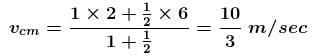## Topic wise Tests for IIT JAM Physics

217 tests
 Use Code STAYHOME200 and get INR 200 additional OFF Use Coupon Code
Information about Centre Of Mass NAT Level - 1 Page
In this test you can find the Exam questions for Centre Of Mass NAT Level - 1 solved & explained in the simplest way possible. Besides giving Questions and answers for Centre Of Mass NAT Level - 1, EduRev gives you an ample number of Online tests for practice

217 tests